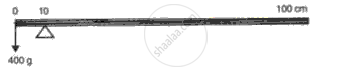Advertisement Remove all ads

# A Meter Scale is Provided at 10 Cm Mark and is Balanced by Suspending 400 G from 0 Cm Mark (Fig. 11). Calculate the Mass of Meter Scale. - Physics

Numerical

A meter scale is provided at 10 cm mark and is balanced by suspending 400 g from 0 cm mark (fig. ). Calculate the mass of meter scale.Advertisement Remove all ads

#### Solution

Let m be the mass of the metre scale

In balancedcondition, sum of clockwise moments = sum of anticlockwise moments

400 x 10 = m x 90

or 4000 = 90m

or m = 44.4g

the mass of the scale is 44.4g

Concept: Moment (Turning Effect) of a Force Or Torque
Is there an error in this question or solution?
Advertisement Remove all ads

#### APPEARS IN

Frank ICSE Class 10 Physics Part 2
Chapter 1 Force, Work, Energy and Power
Exercise | Q 9 | Page 58
Advertisement Remove all ads
Advertisement Remove all ads
Share
Notifications

View all notifications

Forgot password?